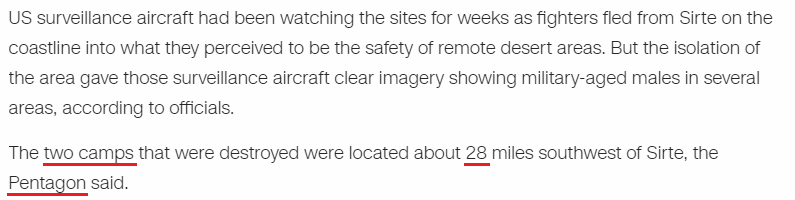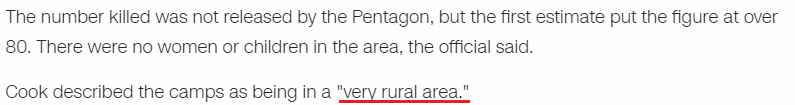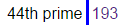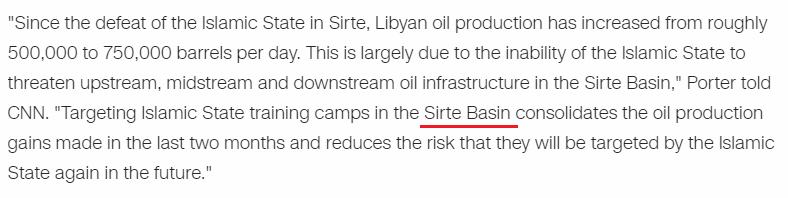CipherJan 20, 2017

CNN: US bombs ISIS camps in Libya (Saturn Worship)

Another classic story by the numbers from CNN http://edition.cnn.com/2017/01/19/politics/us-airstrikes-libya-isis/index.htmlUS bombs ISIS camps = 3 + 1 + 2 + 6 + 4 + 2 + 1 + 9 + 1 + 9 + 1 + 3 + 1 + 4 + 7 + 1 = 55 (Reduced)
dozens killed = 4 + 6 + 8 + 5 + 5 + 1 + 2 + 9 + 3 + 3 + 5 + 4 = 55 (Reduced)

US bombs ISIS camps in Libya, dozens killed = 6+6 +2+2+1+2+6 +5+6+5+6 +3+1+1+3+6 + 5+1 + 2+5+2+2+1 + 4+2+1+5+1+6 +3+5+2+2+5+4 = 119 (Septenary)

Of course this happened on 1/19/2017In the title they say  'dozens killed', and in the post they say the initial estimates are over 80 militants killed
Dozens = 4 + 6 + 8 + 5 + 5 + 10 = 38 (S Exception)
Eighty = 5 + 9 + 7 + 8 + 2 + 7 = 38 (Reduced)
Death = 4 + 5 + 1 + 20 + 8 = 38 (Ordinal)Two camps = 2 + 5 + 6 + 3 + 1 + 4 + 7 + 10 = 38 (S Exception)
Pentagon = 7 + 5 + 5 + 2 + 1 + 7 + 6 + 5 = 38 (Reduced)
Note that they say it was 28 miles southwest of Sirte
28 is 2nd perfect number (7th triangular number)
33 and 28 are the only 2 numbers that sum to 156

Twenty-eight = 20 + 23 + 5 + 14 + 20 + 25 + 5 + 9 + 7 + 8 + 20 = 156 (Ordinal)
Thirty-three = 20 + 8 + 9 + 18 + 20 + 25 + 20 + 8 + 18 + 5 + 5 = 156 (Ordinal)
Pentagon = 60 + 5 + 40 + 100 + 1 + 7 + 50 + 40 = 303 (Jewish)Thirty-three = 2 + 8 + 9 + 9 + 2 + 7 + 2 + 8 + 9 + 5 + 5 = 66 (Reduced)
very rural area = 4 + 5 + 9 + 7 + 9 + 3 + 9 + 1 + 3 + 1 + 9 + 5 + 1 = 66 (Reduced)Spirit bombers = 1 + 7 + 9 + 9 + 9 + 2 + 2 + 6 + 4 + 2 + 5 + 9 + 1 = 66 (Reduced)
Spirit bombers = 100 + 70 + 9 + 90 + 9 + 200 + 2 + 60 + 40 + 2 + 5 + 90 + 100 = 777 (English)
"Cube of Saturn" = 210 + 56 + 511 = 777 (Jewish)
"Order out of Chaos" = 219 + 350 + 56 + 152 = 777 (Jewish)

They always have good names huh?
They were sent from "Whiteman" Air force base
Whiteman = 23 + 8 + 9 + 20 + 5 + 13 + 1 + 14 = 93 (Ordinal)
Whiteman = 900 + 8 + 9 + 100 + 5 + 30 + 1 + 40 = 1093 (Jewish)Also we have "Ash Carter" lying to reportersAsh Carter = 1 + 19 + 8 + 3 + 1 + 18 + 20 + 5 + 18 = 93 (Ordinal)
Saturn = 19 + 1 + 20 + 21 + 18 + 14 = 93 (Ordinal)
B-2 heavy bombers = 2 + 8 + 5 + 1 + 22 + 25 + 2 + 15 + 13 + 2 + 5 + 18 + 19 = 137 (Ordinal)
B-Two Bombers = 2 + 2 + 5 + 6 + 2 + 6 + 4 + 2 + 5 + 9 + 1 = 44 (Reduced)
Kill = 11 + 9 + 12 + 12 = 44 (Ordinal)
Military = 4 + 9 + 3 + 9 + 2 + 1 + 9 + 7 = 44 (Reduced)
Also they are talking to the guy with the last name "Cook" in this post
Cook = 3 + 15 + 15 + 11 = 44 (Ordinal)Sirte basin = 1 + 9 + 9 + 2 + 5 + 2 + 1 + 1 + 9 + 5 = 44 (Reduced)
Genocide = 7 + 5 + 5 + 6 + 3 + 9 + 4 + 5 = 44 (Reduced)
Here is what happened in Lybia on Saturnalia in 2015 (351st day of the year, 26th triangular number is 351, God = 26, Saturn is the God that Jews and secret societies worship mostly)This bombing of ISIS camps happened 33 days after the Roman Holiday Saturnalia
Saturnalia = 10 + 1 + 2 + 3 + 9 + 5 + 1 + 3 + 9 + 1 = 44 (S Exception)

They bombed "Sirte basin" 33 days after Saturnalia, how convenient
Saturnalia = 19 + 1 + 20 + 21 + 18 + 14 + 1 + 12 + 9 + 1 = 116 (Ordinal)
Sirte basin = 19 + 9 + 18 + 20 + 5 + 2 + 1 + 19 + 9 + 14 = 116 (Ordinal)
Entire coding of this story is very Saturnian which is appropriate for this date for many reasons, firstly 1/19 is the where Aquarius and Capricorn meet on the Zodiac, both signs ruled by Saturn, and of course 119 is number very connected to Saturn in gematria
Saturn = 19 + 1 + 20 + 21 + 18 + 14 = 93 (Ordinal)
Saturn = 45 + 1 + 20 + 21 + 18 + 14 = 119 (Francis Bacon)
God Saturn = 7 + 15 + 4 + 19 + 1 + 20 + 21 + 18 + 14 = 119 (Ordinal)
Star of David = 19 + 20 + 1 + 18 + 15 + 6 + 4 + 1 + 22 + 9 + 4 = 119 (Ordinal)
The date when this happened has 21 numerology as well which connects to Saturn as well
1/19/2017 = 1+1+9+2+0+1+7 = 21
Saturn = 1 + 1 + 2 + 3 + 9 + 5 = 21 (Reduced)
It's always about Saturn it seems :)